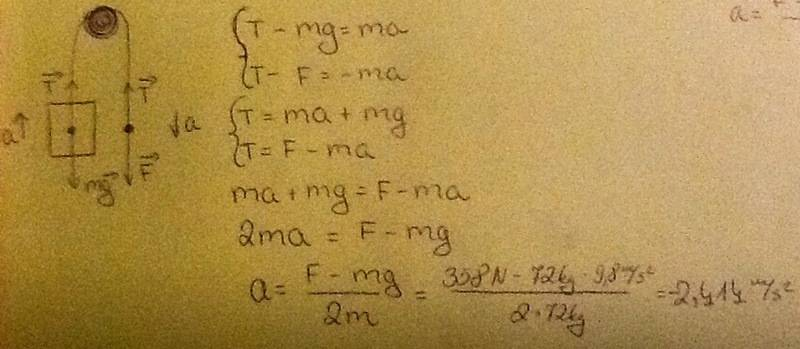# Forces and Newton's Laws

kaspis245

## Homework Statement

To hoist himself into a tree, a 72 kg ties one end of a nylon rope around his waist and throws the other end over a branch of the tree. He then pulls downward on the free end of the rope with a force of 358 N . Neglect amy friction between the rope and the branch, and determine the man's upward acceleration.

## Homework Equations

Newton's laws of motion.

## The Attempt at a SolutionThe answer must be a =0.14m/s2[/B]

## Answers and Replies

How do you get from -2.414m/s2 (handwritten) to 0.14m/s2 (your answer)?
Suppose I changed the problem to: What force must he pull with in order to stay in place (a=0)?

kaspis245
That's easy:
F = mg = 72 kg * 9.8 m/s2 = 705 N

Ben FCB Porter
Firstly:
WMAN = mg = 72x9.8 = 705.6N
So force required to lift the man is 705.6N.

Surely if he pulls on the rope with a force of 358N, he won't be lifted off of the ground at all?

Ben FCB Porter
Firstly:
WMAN = mg = 72x9.8 = 705.6N
So force required to lift the man is 705.6N.

Surely if he pulls on the rope with a force of 358N, he won't be lifted off of the ground at all?

Don't worry, I'm not this stupid, I was mimicking the other commenter hahaha!

kaspis245
I know, but the book gives the answer that a = 0.14 m/s2 . Are you sure it's a mistake?

Ben FCB Porter
I know, but the book gives the answer that a = 0.14 m/s2 . Are you sure it's a mistake?

I believe that because there is a pulley, the question isn't as simple as "he weighs more than the force he exerts so therefore he must not be lifted"; have you learned about the physics of pulleys? I think that is the area of physics this question might be about...

kaspis245
We're dealing with a fixed pulley, so it doesn't change anything.

Ben FCB Porter
I thought the lifting force required is still reduced

Homework Helper
What about newton's 'equal and opposite law'? When the man pulls down on the rope, does the rope not pull back up on him?

What about newton's 'equal and opposite law'? When the man pulls down on the rope, does the rope not pull back up on him?
Yes - but I think the problem lies in the fact that when the man pulls down on the other rope, he does so by transferring some of his "weight" to the other rope. From where else would he get any force?

Homework Helper
Yes - but I think the problem lies in the fact that when the man pulls down on the other rope, he does so by transferring some of his "weight" to the other rope. From where else would he get any force?
You can say it like that if you'd like, but that's saying exactly the same thing. The man pulls down on the rope and the rope pulls up on the man. The rope supports part of the weight of the man, and the weight of the man supported by the rope pulls down on the rope. Etc.

The reason I mention this is because the OP did not include it in his free body diagram.

To the OP:
That's easy:
F = mg = 72 kg * 9.8 m/s2 = 705 N
That's incorrect. Fix your FBD, fix your equations, and try again.

Also, in your equations you wrote, "T-F=-ma" but that "m" is not the same "m" as in the equation above it, is it?